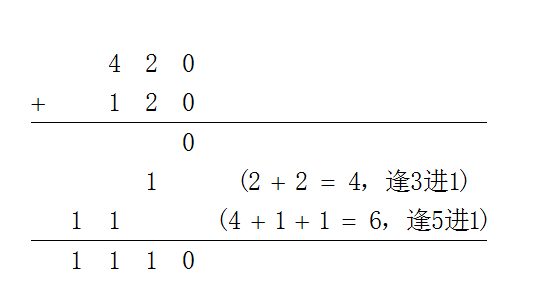# 3057. 素数进制A+B

十进制是逢十进一，十六进制是逢十六进一，二进制就是逢二进一，以此类推，X进制就是逢X进一。

素数进制不是单一进制，在素数进制中，每一位的进制都各不相同，具体定义如下：
+ 将素数从小到大排列成一个素数列表：$2,3,5,7,11,13,17,19,23,29,31,\cdots$
+ 在素数进制表示法中，从右边最低位开始，第$n$位的进制定义为素数列表中第$n$个素数
+ 第$n$位可用的数码为 $0$~$(第n个素数-1)$
+ 第$n$位对应的位权为素数列表中前$n-1$个素数的乘积
+ 第$n$位上的数在运算时逢该素数进一位。

• 十进制整数$2$的素数进制表示为$10$，记为：$1,0$
• 十进制整数$38$的素数进制表示为$1110$，记为：$1,1,1,0$

素数进制表示的两个整数$4,2,0$和$1,2,0$，加法运算的结果为$1,1,1,0$### 样例

Input
3
1,0 2,1
4,2,0 1,2,0
1,0 10,6,4,2,1

Output
case #0:
1,0,1
case #1:
1,1,1,0
case #2:
1,0,0,0,0,1


### 提示

422 人解决，525 人已尝试。

587 份提交通过，共有 2262 份提交。

2.2 EMB 奖励。